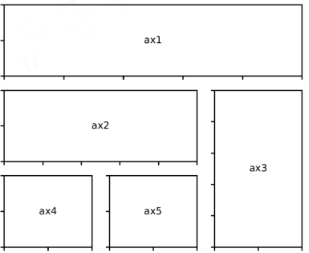# Haste makes waste

[PythonDataAnalysis-XX…]系列，参考Python数据分析与展示 嵩天@北京理工

• 单元1：NumPy库入门：一维、二维、N维、高维数据表示和操作
• 单元2：NumPy数据存取与函数：多维数据存储、随机数函数、统计函数、梯度函数
• 单元3：实战：图像的手绘效果
• 单元4：Matplotlib库的入门和基本使用
• 单元5：Matplotlib基础绘图函数：饼图、直方图、极坐标图、散点图
• 单元6：实战：引力波的绘制
• 单元7：Pandas库入门：Series、DataFrame类型、基本操作
• 单元8：Pandas数据特征分析：数据排序、基本统计分析、累计分析、相关分析

# 1. Matplotlib库的使用

Matplotlib库由各种可视化类构成，内部结构复杂，受Matlab启发 matplotlib.pyplot是绘制各类可视化图形的命令子库，相当于快捷方式。

import matplotlib.pyplot as plt

plt.plot([3,1,4,5,2])
plt.savefig('test.jpg',dpi=600) # 以PNG格式保存,dpi控制输出质量
plt.show()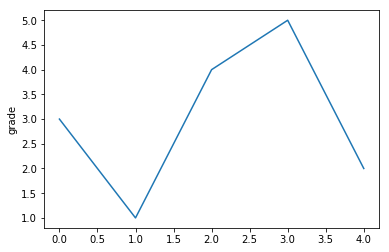• plt.plot()只有一个输入列表或数组时，参数被当作Y轴，X轴以索引自动生成。
• plt.plot(x,y)当有两个以上参数时，按照X轴和Y轴顺序绘制数据点。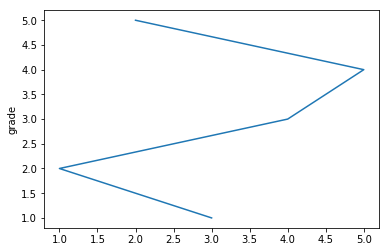# 2. 关于绘图区域

• plt.subplot(nrows, ncols, plot_number) 例如 plt.subplot(3,2,4) plt.subplot(324)
• 在全局绘图区域中创建一个分区体系，并定位到一个子绘图区域 。上面的代码中创建了一个3行2列的区域，当前绘图区域为第4部分。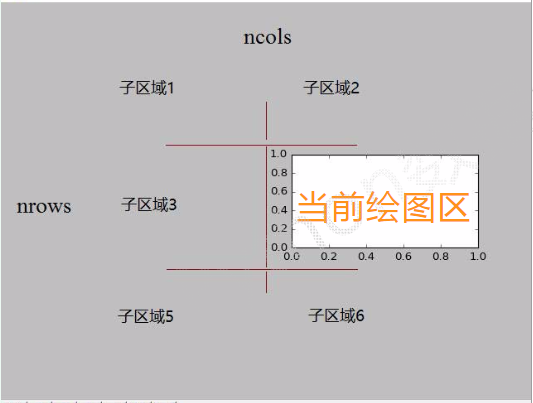• 示例代码
import matplotlib.pyplot as plt
import numpy as np

def f(t):
return np.exp(-t) * np.cos(2*np.pi*t)

# 以0.02为间隔，从0到5之间取点，一共250个点
a = np.arange(0.0,5.0,0.02)

# 在2行一列中的第一区域绘图
plt.subplot(211)
plt.plot(a,f(a))

# 在2行一列中的第二区域绘图
plt.subplot(2,1,2)
# r--表示绘图线形
plt.plot(a,np.cos(2*np.pi*a),'r--')

plt.show()


exp函数是求指数的意思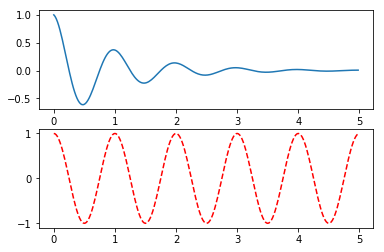# 3. plot()函数

• plt.plot(x, y, format_string, **kwargs)
1. x : X轴数据，列表或数组，可选
2. y :Y 轴数据，列表或数
3. format_string: 控制曲线的格式字符串，可选. 由颜色字符风格字符标记字符组成.
4. **kwargs : 第二组或更多(x,y,format_string)

## 3.1 format_string 详解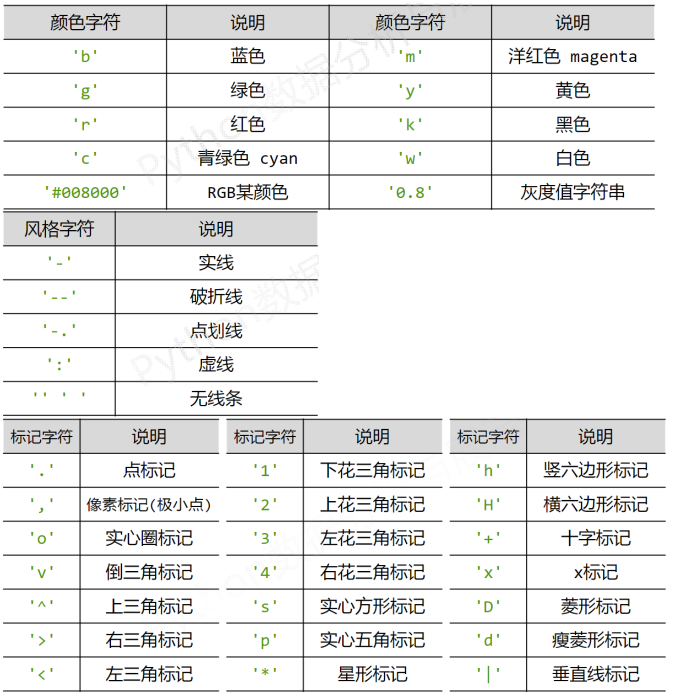import matplotlib.pyplot as plt
import numpy as np

a = np.arange(10)
plt.plot(a,a*1.5,'go-',a,a*2.5,'rx',a,a*3.5,'*',a,a*4.5,'b-.')

plt.show()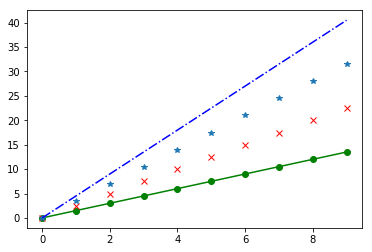# 4. pyplot中的中文显示

## 4.1 中文显示方法1

import matplotlib.pyplot as plt

# 追加了声明
plt.rcParams['font.family'] = 'Simhei'
plt.plot([3,1,4,5,2])
plt.ylabel('纵轴(值)')
plt.savefig('test.jpg',dpi=600) # 以PNG格式保存,dpi控制输出质量
plt.show()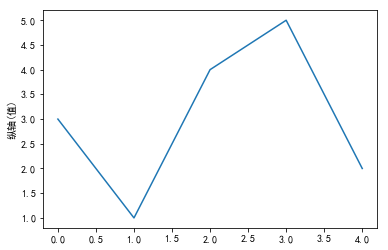• ‘font.family’ 用于显示字体的名字
• ‘font.style’ 字体风格，正常’normal’或斜体’italic’
• ‘font.size’字体大小，整数字号或者’large’、’x‐small’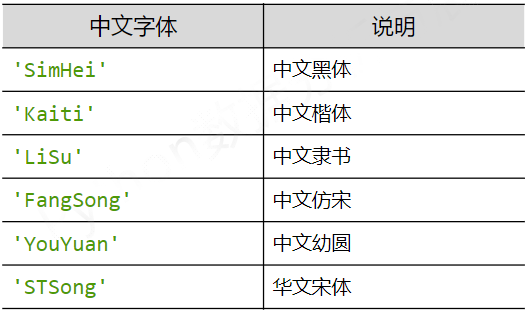## 4.2 中文显示方法2

import matplotlib.pyplot as plt

plt.rcParams['font.family'] = 'Simhei'
plt.plot([3,1,4,5,2])
plt.ylabel('纵轴(值)',fontproperties='SimHei',fontsize=20)
plt.xlabel('横轴(值)',fontproperties='SimHeiSimHei',fontsize=10)
plt.savefig('test.jpg',dpi=600) # 以PNG格式保存,dpi控制输出质量
plt.show()



X和Y方向的字大小不一致，如下图: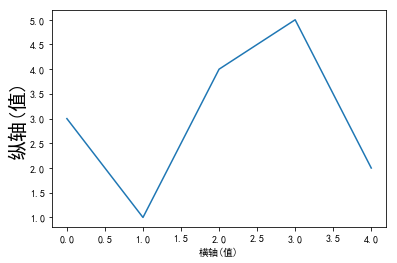# 5. pyplot的文本显示函数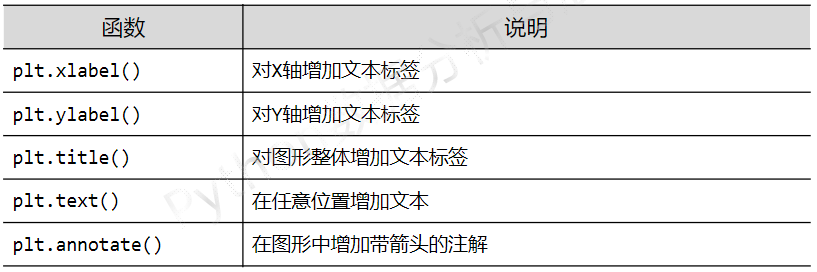import matplotlib.pyplot as plt
import numpy as np

a = np.arange(0.0,5.0,0.02)
plt.plot(a,np.cos(2*np.pi*a),'r--')
plt.ylabel('纵轴(值)',fontproperties='SimHei',fontsize=20,color='green')
plt.xlabel('横轴(值)',fontproperties='SimHei',fontsize=20)

# $是一种Latex语法 plt.title(r'正弦波示例$y=cos(2\pi x)$',fontproperties='SimHei',fontsize=25) plt.text(2,1,r'$\mu=100$',fontsize=15) # 使数字前的负号能正确显示 plt.rcParams['axes.unicode_minus'] = False plt.axis([-1,6,-2,2]) plt.grid(True) plt.show()  显示图像如下: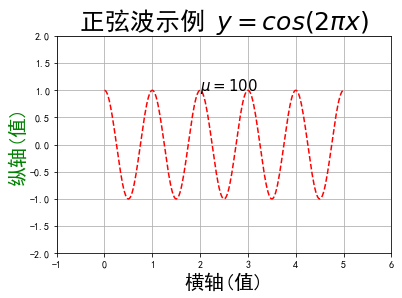还可以显示标注，如将上面的text函数部分修改为如下后 plt.annotate(r'$\mu=100\$',xy=(2,1),xytext=(3,1.5),arrowprops=dict(facecolor='blue',shrink=0.1,width=2))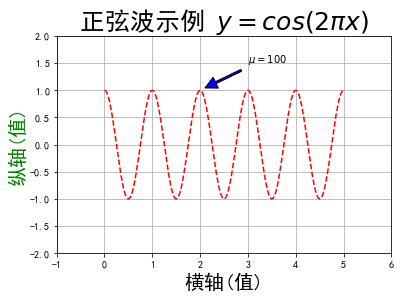# 6. pyplot的复杂子绘图区域